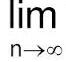29 - Limit Continuity and Differentiability Questions Answers

SCRA 2013 SET D, QUES. 10( [x]+[2x]+[3x]+.....[nx] ) /  n2

Asked By: GAUTAM SAGAR 5 year agoAsked By: AKHIL CHAND 5 year ago

all are defined

put π in the first, factorise second and multiply the conjugate of numerator in the thirdAsked By: AKHIL CHAND 5 year ago

factorise numerator and denominator

show that limit /x/ = 0 as x approaches 0.

Asked By: FAITH MWONGELI 6 year ago

show that limit /x/ = 0 as x approaches 0.

Asked By: FAITH MWONGELI 6 year ago

if f is a real valued differentiable function satisfying If(x) - f(y)I ≤ (x-y)2   x,yεR and f(0) =0 then f(1) equals

(a) 1

(b) 2

(c) 0

(d) -1

Asked By: HIMANSHU MITTAL 6 year agoSolved By: VISHAL PHOGAT

let g(x) be the inverse of the function f(x), and f/(x) = 1/1+x3 then g/(x) equals

(1) 1/1+g3

(2) 1/1+f3

(3) 1+ g3

(4) 1+f3

Asked By: HIMANSHU MITTAL 6 year agoSolved By: VISHAL PHOGAT

let tr = r/1+r2+ r4  then lim n-->∑ tr

Asked By: HIMANSHU MITTAL 6 year ago

lim n-->∞ [tanx + 1/2tan(x/2) + 1/22tan(x/22) + ............. + 1/2ntan(x/2n)]

Asked By: HIMANSHU MITTAL 6 year ago